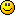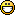# forex software

Create and Test Forex Strategies

forex software

Forex Forum

Forex Software, Forex Strategies, Expert Advisors Generator

# Filter indicator

Forex Forum → Filter indicator

Pages 1

## Posts: 14

#### Topic: Filter indicator

Dear all i opened this topic for digital filters requests and sharing thoughts about them

SATL and FATL

SATL indicator

#### Re: Filter indicator

Here some sources shows the filters

http://www.forex-tsd.com/digital-filters/76-digital-filters-basic-explanation.html

#### Re: Filter indicator

Ahmed, find Fatl&Satl here: http://forexsb.com/forum/post/15959/#p15959.

#### Re: Filter indicator

thanks for fast response#### Re: Filter indicator

ahmedalhoseny wrote:

thanks for fast responseThey were pretty easy. I was thinking maybe I should combine them in a crossover indi, what do you think?

it will be fine

#### Re: Filter indicator

i donot understand the concept behind the filter indicators calculations they look like MAs but sure they arenot

and you already coded them so footon could you teel me how you read these indicators and how the indicator links between the arrays " the down part of indicator" with the values in the upper part

Regards

#### Re: Filter indicator

Please explain more, I'm not understanding the question I'm afraid.

#### Re: Filter indicator

WAW
you completely changed the code
when iam looking to the 1st satl and compare to new satl you modified  some parts of code
/*// The NumericUpDown parameters
IndParam.NumParam.Caption   = "period";
IndParam.NumParam.Value     = 80;
IndParam.NumParam.Min       = 2;
IndParam.NumParam.Max       = 200;
//IndParam.NumParam.Point     = 1;
IndParam.NumParam.Enabled   = true;
IndParam.NumParam.ToolTip   = "The Moving Average period.";

/*IndParam.NumParam.Caption   = "ShortPeriod";
IndParam.NumParam.Value     = 8.0;
IndParam.NumParam.Min       = 0.1;
IndParam.NumParam.Max       = 200;
IndParam.NumParam.Point     = 1;
IndParam.NumParam.Enabled   = true;
IndParam.NumParam.ToolTip   = "ShortPeriod";

IndParam.NumParam.Caption   = "ExtraTimeForward";
IndParam.NumParam.Value     = 1.0;
IndParam.NumParam.Min       = 0;
IndParam.NumParam.Max       = 200;
IndParam.NumParam.Point     = 1;
IndParam.NumParam.Enabled   = true;
IndParam.NumParam.ToolTip   = "ExtraTimeForward";*/

/*IndParam.NumParam.Caption   = "deviation";
IndParam.NumParam.Value     = 0;
IndParam.NumParam.Min       = 0;
IndParam.NumParam.Max       = 200;
IndParam.NumParam.Enabled   = true;
IndParam.NumParam.ToolTip   = "How many bars to shift with.";*/

I get lost !!!!!!!

#### Re: Filter indicator

In SATL V2  what is the use of this part of code !!!!!!!

// The NumericUpDown parameters
/*IndParam.NumParam.Caption = "Period";
IndParam.NumParam.Value = 14;
IndParam.NumParam.Min = 1;
IndParam.NumParam.Max = 200;
IndParam.NumParam.Enabled = true;
IndParam.NumParam.ToolTip = "The Moving Average period.";

IndParam.NumParam.Caption = "Shift";
IndParam.NumParam.Value = 0;
IndParam.NumParam.Min = 0;
IndParam.NumParam.Max = 200;
IndParam.NumParam.Enabled = true;
IndParam.NumParam.ToolTip = "How many bars to shift with.";*/

#### Re: Filter indicatorCalculation did not change, and top tip: /* */   means it is commented out of the code, it is not used.

This is the calculation block:

``````SATLBuffer[iBar]=

What is between /* */ is NOT important. I changed the base code for getting rid of the bug fast.

#### Re: Filter indicator

AHA
I  get it Now

Fotton i have an idea as soon as fatl and satl using the same way of calculation is that better to make the calculation block " buffer" universal by breaking the code to its parts and make the indicator dynamic in selecting which combinations of buffers !!!!

Lets take an example

Part 1- "- Nums---- +Nums "
Part 3- iBar-0.........iBar200
Part4- how many Lines it will select to build the satl

It may
.
.
.

it may
.
.
.

Is that applicable

#### Re: Filter indicator

It should be possible, but I have to think about it a lot to figure the solution out.

Pages 1